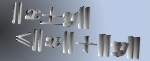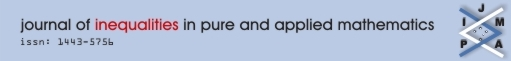Home Editors Submissions Reviews Volumes RGMIA About Us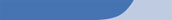Volume 2, Issue 3, Article 29

A Weighted Analytic Center for Linear Matrix Inequalities

Authors: Irwin S. Pressman, Shafiu Jibrin,
Keywords: Weighted analytic center, Semidefinite Programming, Linear Matrix Inequalities, Convexity, Real Algebraic Variety.
Date Accepted: 21/03/01
Subject Codes:

90C25,49Q99,46C05,14P25

Editors: Jonathan Borwein,

Abstract:

Let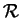be the convex subset of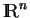defined by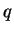simultaneous linear matrix inequalities (LMI)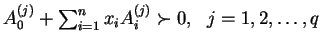. Given a strictly positive vector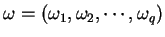, the weighted analytic center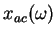is the minimizerargmin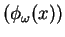of the strictly convex function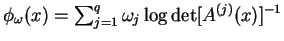over. We give a necessary and sufficient condition for a point ofto be a weighted analytic center. We study the argmin function in this instance and show that it is a continuously differentiable open function.

In the special case of linear constraints, all interior points are weighted analytic centers. We show that the region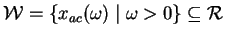of weighted analytic centers for LMI's is not convex and does not generally equal. These results imply that the techniques in linear programming of following paths of analytic centers may require special consideration when extended to semidefinite programming. We show that the region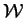and its boundary are described by real algebraic varieties, and provide slices of a non-trivial real algebraic variety to show thatisn't convex. Stiemke's Theorem of the alternative provides a practical test of whether a point is in. Weighted analytic centers are used to improve the location of standing points for the Stand and Hit method of identifying necessary LMI constraints in semidefinite programming.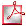Download Screen PDFDownload Print PDF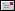Send this article to a friend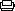Print this pagesearch [advanced search]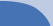copyright 2003terms and conditions login